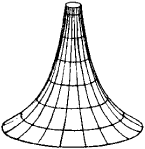# Pseudosphere

Also found in: Dictionary, Wikipedia.

## pseudosphere

[′süd·ə‚sfir]
(mathematics)
The pseudospherical surface generated by revolving a tractrix about its asymptote.
McGraw-Hill Dictionary of Scientific & Technical Terms, 6E, Copyright © 2003 by The McGraw-Hill Companies, Inc.
The following article is from The Great Soviet Encyclopedia (1979). It might be outdated or ideologically biased.

## Pseudosphere

the surface of constant negative curvature formed by the rotation of a tractrix about its asymptote (see Figure 1). Its name emphasizes both its similarity to and difference from a sphere, which is an example of a surface with constant positive curvature. The pseudosphere is of particular interest because figures drawn on smooth parts of this surfaceobey the laws of Lobachevskian non-Euclidean geometry. This fact, established in 1868 by E. Beltrami, was of great importance in the dispute over the reality of Lobachevskian geometry.

The Great Soviet Encyclopedia, 3rd Edition (1970-1979). © 2010 The Gale Group, Inc. All rights reserved.
References in periodicals archive ?
Caption: Figure 3: Upper half of a tractricoid (pseudosphere).
The origin of [S.sub.(2)] is O = (1, 0, 0, 0, 0, 0) and this space is identified with the intersection of the pseudosphere [[summation].sub.(2)] determined by [I.sub.(2)] with a quadratic cone P known as Plucker or Grassmann relation (invariant under the group action); these constraints are given by 
Then, by using a perturbation method, we were able to analytically calculate the shape of the buckled membrane and show that it is a pseudosphere.
Defects in nematic membranes can buckle into pseudospheres. Physical Review E, v.
In this paper we define nonnull and null pseudospherical Smarandache curves according to the Sabban frame of a spacelike curve lying on pseudosphere in Minkowski 3-space.
The pseudosphere with center at the origin and of radius r = 1 in the Minkowski 3-space [R.sup.3.sub.1] is a quadric defined by
Let [alpha] : I [subset] R [right arrow] [S.sup.2.sub.1] be a curve lying fully in pseudosphere [S.sup.2.sub.1] in [R.sup.3.sub.1].
Example 7 (the de Sitter pseudosphere is shown in Figure 6).
that is, the de Sitter pseudosphere satisfies condition (3).
Then a is congruent to an osculating curve of the second kind with tangential component g([alpha], T) = 0 if and only if [alpha] lies in a pseudosphere [S.sup.3.sub.1](r) in [E.sup.4.sub.1].
Hence a is a null helix lying in the pseudosphere. According to Theorem 4.4, [alpha] is an osculating curve of the second kind.
It is then clear that all complexified Heisenberg (k, n - k) pseudospheres

Site: Follow: Share:
Open / Close